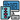# Why do we need Algorithms?

To properly learn something you have to start from the beginning. In this series of articles, We(yes me too) will learn about algorithms step by step. There are many books, tutorials, and blogs available on Algorithms, and it is often confusing to choose anyone from this large pool of resources. I was also confused but now I have decided that I will provide a summary of all the resources available and people don't have to look in different websites for every different topic.

This article, and the possible series I'll create from it, is meant for those who want to learn algorithms in an efficient way step by step and from a single resource or looking to revise concepts before a coding interview.

#### Goals

The goal of this article is to focus on understanding the basic elements of algorithms, the importance of algorithm analysis, and then move toward the other topics as mentioned here.

So, let's begin 🚀

##### Data Types

Let's take an example of a mathematical equation:

``````// Equation of Circle
x^2 + y^2 = 1``````

We have two names `x` and `y` which holds the value(data), which means they are placeholders for representing data. In Computer science, we need something for holding data, and variables is the way to do that.

To find a solution to this equation, we need to know the type of values that they can take, like integers, real numbers, complex numbers, etc. Data type is the term used in computer science for this purpose. Example: integer, float, character, string, etc.

• Why we have data types in the programming language?

Computer memory is zeros and ones. Imagine how difficult it will be if you want to code in terms of zeros and ones. To help, programming languages and compilers provide us with data types.

• What are the different data types?

There are two types of data types at top level:

• System defined(Primitive) data types: `int`, `float`, `char`, `str` etc.
• User defined data types: `struct`, `class`
##### Data Structures

Now moving ahead, we have the equation and we want to solve it. We have data in variables and now require some mechanism to solve the equation. Data Structure is a way of storing and organizing data in a computer to use it efficiently. Example: `arrays`, `files`, `stack`, `queues`, `trees` etc.

• How data structures is classified?

Based on organizing the data, it is of two types:

• Linear Data Structures - Data accessed in sequential order but may/may not be stored sequentially. Example: `linked lists`, `stacks` & `queues`
• Non-Linear Data Structures - Data accessed/stored in a non-linear order. Example: Trees and Graphs
• What is Abstract Data Types(ADTs)?

• To simplify the process of solving the equation, data structures are combined with their operations which are known as ADTs. It has two parts:

• Declaration of data
• Declaration of operations
##### What is an Algorithm?

In simple terms, a step-by-step procedure for solving a given problem is known as an algorithm. Formally,

An algorithm is an explicit, precise, unambiguous, mechanically-executable the sequence of elementary instructions usually intended to accomplish a specific purpose.

We judge the algorithms by its correctness and efficiency(in terms of memory and time).

##### Why the Analysis of Algorithm?

In computer science or in general, also, there are many ways to solve a given problem. Analysis of these ways(here algorithms) helps to determine which is most efficient in terms of time and space(memory) consumed. It also includes other factors such as developer effort etc.

Now, you must have the basic idea of how we are going to process further. Do not memorize these things and just understand the underlying concepts.

In the upcoming articles, we will discuss different ways of analysis of algorithms and the most important Big-O notation and much more.

Here are the next articles on Algorithms.

##### Did you find this post useful?

I would be really grateful if you let me know by sharing it on Twitter!

Follow me @ParthS0007 for more tech and blogging content :)